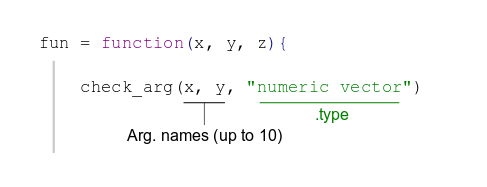This package aims to improve the user experience of R-users (just that! :-)). It offers developers, or anyone writing functions (therefore everyone!), a simple, flexible and powerful way to check all arguments passed to a function.

Debugging a call to a function can be a very frustrating experience: misleading and unclear error messages can make it quite long to finally make the function work. But this needs not be the case. The `dreamerr` package provides the `check_arg` function that does all the work of checking the arguments for you. From the developer side, it is easy and intuitive to perform a full check on the arguments passed to the function: in one line of code. From the user side, they will get, if they provide wrong arguments, useful and informative error messages. In terms of perfomance, since the code has been highly optimized, the cost of checking arguments with `check_arg` is close to 0.

This short introduction gives some basics on how to use `check_arg` after the following example.

# Motivating example

You have two numeric vectors x and y and you want to compute `sum(x, y)`:

``sum(x, y)``
``## Error in sum(x, y): invalid 'type' (closure) of argument``

Bam! there’s a problem… If you’re new to R, this message will look quite puzzling–and very unlikely to be helpful. Even if you’re an experienced R user (who’re acquainted to such error messages), it’s impossible to understand from where the problem comes from.

Now you run the same function but with error-handling:

``sum_check(x, y)``
``````## Error: in sum_check(x, y):
##  In argument '...', each element must be a numeric vector. Problem in
## the second element: it is not a vector, it's a function.``````

It’s still an error. But now anyone can clearly understand the problem (even if you’re a R-beginner)! It tells us that what we thought was a vector (`y`) was in fact a function. The time to debug this error is 0s due to the clarity of the error message: we know which argument is the culprit and we know exactly what the problem is.

There’s a big gap in terms of user experience between the two functions, although there’s only one line of code of difference between the two:

``````sum_check = function(...){
check_arg(..., "numeric vector")
sum(...)
}``````

# How to use `check_arg`

The package offers (almost only) a single function: `check_arg`. This function must be placed within a function, its arguments must be argument names (the ones of the function), followed by the type these arguments are expected to have. As follows:Here are detailed only the basics. For much more detailed information, with numerous examples, please see the help page of `check_arg`.

### Argument `.type`

The `.type` argument is the big thing. It supports literally hundreds of different types and uses natural language to express them. It must be a single character string, composed of several types each separated by a pipe (`|`). For example if we use `"logical scalar | ts formula | data.frame ncol(,2)"`, it means that the argument must be either: a logical scalar (i.e. `TRUE` or `FALSE`), ii) a two-sided formula, or iii) a data.frame with maximum 2 columns. Each pipe separates a type, each type is equal to one main class (here `scalar`, `formula` and `data.frame`) with (optionally) additional restrictions (here `logical`, `ts` and `ncol(,2)`). The table below shows the 13 different class codes and the restrictions they are associated to.